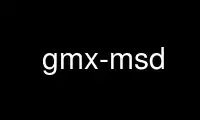# gmx-msd - Online in the CloudThis is the command gmx-msd that can be run in the OnWorks free hosting provider using one of our multiple free online workstations such as Ubuntu Online, Fedora Online, Windows online emulator or MAC OS online emulator

### PROGRAM:

#### NAME

gmx-msd - Calculates mean square displacements

#### SYNOPSIS

gmx msd [-f [<.xtc/.trr/...>]] [-s [<.tpr/.gro/...>]] [-n [<.ndx>]]
[-o [<.xvg>]] [-mol [<.xvg>]] [-pdb [<.pdb>]] [-b <time>]
[-e <time>] [-tu <enum>] [-[no]w] [-xvg <enum>]
[-type <enum>] [-lateral <enum>] [-[no]ten] [-ngroup <int>]
[-[no]mw] [-[no]rmcomm] [-tpdb <time>] [-trestart <time>]
[-beginfit <time>] [-endfit <time>]

#### DESCRIPTION

gmx msd computes the mean square displacement (MSD) of atoms from a set of initial
positions. This provides an easy way to compute the diffusion constant using the Einstein
relation. The time between the reference points for the MSD calculation is set with
-trestart. The diffusion constant is calculated by least squares fitting a straight line
(D*t + c) through the MSD(t) from -beginfit to -endfit (note that t is time from the
reference positions, not simulation time). An error estimate given, which is the
difference of the diffusion coefficients obtained from fits over the two halves of the fit
interval.

There are three, mutually exclusive, options to determine different types of mean square
displacement: -type, -lateral and -ten. Option -ten writes the full MSD tensor for each
group, the order in the output is: trace xx yy zz yx zx zy.

If -mol is set, gmx msd plots the MSD for individual molecules (including making molecules
whole across periodic boundaries): for each individual molecule a diffusion constant is
computed for its center of mass. The chosen index group will be split into molecules.

The default way to calculate a MSD is by using mass-weighted averages. This can be turned
off with -nomw.

With the option -rmcomm, the center of mass motion of a specific group can be removed. For
trajectories produced with GROMACS this is usually not necessary, as gmx mdrun usually
already removes the center of mass motion. When you use this option be sure that the
whole system is stored in the trajectory file.

The diffusion coefficient is determined by linear regression of the MSD, where, unlike for
the normal output of D, the times are weighted according to the number of reference
points, i.e. short times have a higher weight. Also when -beginfit``=-1,fitting starts at
10% and when ``-endfit``=-1, fitting goes to 90%. Using this option one also gets an
accurate error estimate based on the statistics between individual molecules. Note that
this diffusion coefficient and error estimate are only accurate when the MSD is completely
linear between ``-beginfit and -endfit.

Option -pdb writes a .pdb file with the coordinates of the frame at time -tpdb with in the
B-factor field the square root of the diffusion coefficient of the molecule. This option
implies option -mol.

#### OPTIONS

Options to specify input files:

-f [<.xtc/.trr/...>] (traj.xtc)
Trajectory: xtc trr cpt gro g96 pdb tng

-s [<.tpr/.gro/...>] (topol.tpr)
Structure+mass(db): tpr gro g96 pdb brk ent

-n [<.ndx>] (index.ndx) (Optional)
Index file

Options to specify output files:

-o [<.xvg>] (msd.xvg)
xvgr/xmgr file

-mol [<.xvg>] (diff_mol.xvg) (Optional)
xvgr/xmgr file

-pdb [<.pdb>] (diff_mol.pdb) (Optional)
Protein data bank file

Other options:

-b <time> (0)
First frame (ps) to read from trajectory

-e <time> (0)
Last frame (ps) to read from trajectory

-tu <enum> (ps)
Unit for time values: fs, ps, ns, us, ms, s

-[no]w (no)
View output .xvg, .xpm, .eps and .pdb files

-xvg <enum>
xvg plot formatting: xmgrace, xmgr, none

-type <enum> (no)
Compute diffusion coefficient in one direction: no, x, y, z

-lateral <enum> (no)
Calculate the lateral diffusion in a plane perpendicular to: no, x, y, z

-[no]ten (no)
Calculate the full tensor

-ngroup <int> (1)
Number of groups to calculate MSD for

-[no]mw (yes)
Mass weighted MSD

-[no]rmcomm (no)
Remove center of mass motion

-tpdb <time> (0)
The frame to use for option -pdb (ps)

-trestart <time> (10)
Time between restarting points in trajectory (ps)

-beginfit <time> (-1)
Start time for fitting the MSD (ps), -1 is 10%

-endfit <time> (-1)
End time for fitting the MSD (ps), -1 is 90%

Use gmx-msd online using onworks.net services

Free Servers & Workstations